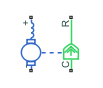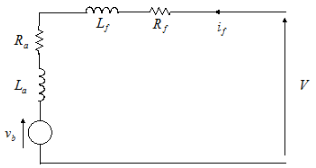# Universal Motor

Universal (or series) motor with electrical and torque characteristics

• Library:
• Simscape / Electrical / Electromechanical / Brushed Motors

•## Description

The Universal Motor block represents the electrical and torque characteristics of a universal (or series) motor using the following equivalent circuit model.Where:

• Ra is the armature resistance.

• La is the armature inductance.

• Rf is the field winding resistance.

• Lf is the field winding inductance.

When you set the Model parameterization parameter to ```By equivalent circuit parameters```, you specify the equivalent circuit parameters for this model. The Universal Motor block computes the motor torque as follows:

1. The magnetic field in the motor induces the following back emf vb in the armature:

`${v}_{b}={L}_{af}{i}_{f}\omega$`

where Laf is a constant of proportionality and ω is the angular velocity.

2. The mechanical power is equal to the power reacted by the back emf:

`$P={v}_{b}{i}_{f}={L}_{af}{i}_{f}{}^{2}\omega$`

3. The motor torque is:

`$T=P/\omega ={L}_{af}{i}_{f}{}^{2}$`

The torque-speed characteristic for the Universal Motor block model is related to the parameters in the preceding figure. When you set the Model parameterization parameter to ```By DC rated power, rated speed & maximum torque``` or ```By DC rated power, rated speed & electrical power```, the block solves for the equivalent circuit parameters as follows:

1. For the steady-state torque-speed relationship when using a DC supply, L has no effect.

2. Sum the voltages around the loop:

`$V=\left({R}_{f}+{R}_{a}\right){i}_{f}+{v}_{b}=\left({R}_{f}+{R}_{a}+{L}_{af}\omega \right){i}_{f}$`

3. Solve the preceding equation for if and substitute this value into the equation for torque:

`$T={L}_{af}{\left(\frac{V}{{R}_{f}+{R}_{a}+{L}_{af}\omega }\right)}^{2}$`

The block uses the rated speed and power to calculate the rated torque. The block uses the rated torque and rated speed values in the preceding equation plus the corresponding electrical power to determine values for Rf+Ra and Laf.

When you set the Model parameterization parameter to ```By AC rated power, rated speed, current & electrical power```, then the block must include the inductive terms La and Lf in the model. This requires information about the RMS rated current and voltage for the total inductance.

The block models motor inertia J and damping B for all values of the Model parameterization parameter. The output torque is:

`${T}_{load}={L}_{af}{\left(\frac{V}{{R}_{f}+{R}_{a}+{L}_{af}\omega }\right)}^{2}-J\stackrel{˙}{\omega }-B\omega$`

The block produces a positive torque acting from the mechanical C to R ports.

### Model Thermal Effects

You can expose thermal ports to model the effects of losses that convert power to heat. To expose the thermal ports, set the Modeling option parameter to either:

• `No thermal port` — The block does not contain thermal ports.

• `Show thermal port` — The block contains multiple thermal conserving ports.

For more information about using thermal ports in actuator blocks, see Simulating Thermal Effects in Rotational and Translational Actuators.

## Ports

### Conserving

expand all

Electrical conserving port associated with the universal motor positive terminal.

Electrical conserving port associated with the universal motor negative terminal.

Mechanical rotational conserving port associated with the universal motor case.

Mechanical rotational conserving port associated with the universal motor rotor.

Field winding thermal port.

#### Dependencies

To enable this port, set Modeling option to ```Show thermal port```.

Armature winding thermal port.

#### Dependencies

To enable this port, set Modeling option to ```Show thermal port```.

## Parameters

expand all

Whether to enable the thermal ports of the block and model the effects of losses that convert power to heat.

### Electrical Torque

Select one of the following methods for block parameterization:

• `By equivalent circuit parameters` — Provide electrical parameters for an equivalent circuit model of the motor.

• `By DC rated power, rated speed & maximum torque` — Provide DC power and speed parameters that the block converts to an equivalent circuit model of the motor.

• `By DC rated power, rated speed & electrical power` — Provide AC power and speed parameters that the block converts to an equivalent circuit model of the motor.

• ```By AC rated power, rated speed, current & electrical power``` — Provide AC power and speed parameters that the block converts to an equivalent circuit model of the motor.

Total resistance of the armature and field winding.

#### Dependencies

This parameter is visible only when you set the Model parameterization parameter to ```By equivalent circuit parameters```.

The ratio of the voltage generated by the motor to the motor speed.

#### Dependencies

This parameter is visible only when you set the Model parameterization parameter to ```By equivalent circuit parameters```.

Total inductance of the armature and field winding. If you do not have information about this inductance, set the value of this parameter to a small, nonzero number.

Note

You can set the Total armature and field winding inductance value to zero, but this only makes sense if you are driving the motor with a DC source.

#### Dependencies

This parameter is visible only when you set the Model parameterization parameter to ```By equivalent circuit parameters```, ```By DC rated power, rated speed & maximum torque```, or ```By DC rated power, rated speed & electrical power```.

Motor speed at the rated mechanical load.

#### Dependencies

This parameter is visible only when you set the Model parameterization parameter to ```By DC rated power, rated speed & maximum torque```, ```By DC rated power, rated speed & electrical power```, or ```By AC rated power, rated speed, current & electrical power```.

The mechanical load for which the motor is rated to operate.

#### Dependencies

This parameter is visible only when you set the Model parameterization parameter to ```By DC rated power, rated speed & maximum torque```, ```By DC rated power, rated speed & electrical power```, or ```By AC rated power, rated speed, current & electrical power```.

The DC voltage at which the motor is rated to operate.

#### Dependencies

This parameter is visible only when you set the Model parameterization parameter to ```By DC rated power, rated speed & maximum torque``` or ```By DC rated power, rated speed & electrical power```.

Maximum torque the motor produces.

#### Dependencies

This parameter is visible only when you set the Model parameterization parameter to ```By DC rated power, rated speed & maximum torque```.

The amount of electrical power the motor uses at the rated mechanical power.

#### Dependencies

This parameter is visible only when you set the Model parameterization parameter to ```By DC rated power, rated speed & electrical power``` or ```By AC rated power, rated speed, current & electrical power```.

RMS supply voltage when the motor operates on AC power.

#### Dependencies

This parameter is visible only when you set the Model parameterization parameter to ```By AC rated power, rated speed, current & electrical power```.

RMS current when the motor operates on AC power at the rated load.

#### Dependencies

This parameter is visible only when you set the Model parameterization parameter to ```By AC rated power, rated speed, current & electrical power```.

Frequency of the AC supply voltage.

#### Dependencies

This parameter is visible only when you set the Model parameterization parameter to ```By AC rated power, rated speed, current & electrical power```.

### Mechanical

Resistance of the rotor to change in motor motion. The value can be zero.

Energy dissipated by the rotor. The value can be zero.

Speed of the rotor at the start of the simulation.

### Temperature Dependence

This tab appears only for blocks with exposed thermal ports.

The ratio of the field to the armature resistance. This parameter is required only when showing the field and armature thermal ports. It is used to determine individual resistance values for the field and armature windings so that the thermal heat generated by the two resistors can be apportioned correctly.

A 1 by 2 row vector that defines the coefficient α in the equation relating resistance to temperature, as described in Thermal Model for Actuator Blocks. The first element corresponds to the field winding, and the second to the armature. The default value is for copper.

The temperature for which motor parameters are defined.

### Thermal Port

This tab appears only for blocks with exposed thermal ports.

A 1 by 2 row vector that defines the thermal mass for the field and armature windings. The thermal mass is the energy required to raise the temperature by one degree.

A 1 by 2 row vector that defines the temperature of the field and armature thermal ports at the start of simulation.

 Bolton, W. Mechatronics: Electronic Control Systems in Mechanical and Electrical Engineering, 3rd edition Pearson Education, 2004..